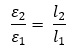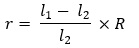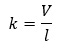A potentiometer is a device used to measure an unknown emf or potential difference accurately.

The potentiometer is a device to compare potential differences. Since the method involves a condition of no current flow, the device can be used to measure potential difference; internal resistance of a cell and compare emf’s of two sources.

This is a versatile instrument. It is basically a long piece of uniform wire, sometimes a few meters in length across which a standard cell is connected. In actual design, the wire is sometimes cut in several pieces placed side by side and connected at the ends by thick metal strip..

A current I flows through the wire which can be varied by a variable resistance (rheostat, R) in the circuit. Since the wire is uniform, the potential difference between A and any point at a distance l from A iswhere ϕ is the potential drop per unit length.Fig. A potentiometer. G is a galvanometer and R a variable resistance (rheostat). 1, 2, 3 are terminals of a two way key
(a) circuit for comparing emfs of two cells; (b) circuit for determining internal resistance of a cell.

### Some Important point about Potentiometer:

• A potentiometer can be regarded as an ideal voltmeter with infinite resistance because it does not draw any current from the source of emf at the null point.
• The principle of potentiometer requires that (i) the potentiometer wire should be of uniform cross-section and (ii) the current through the wire should remain constant.
• The emf of the auxiliary battery must be greater than the emf of the cell to be measured.
• The balance point cannot be obtained on the potentiometer if the fall of potential along the potentiometer wire due to the auxiliary battery is less than the emf of the cell to be measured.
• The positive terminals of the auxiliary battery and the cell whose emf is to be determined must be connected to the zero end of the potentiometer.

Other uses of a potentiometer: Any physical quantity that can produce or control a potential difference can be measured using a potentiometer. Thus, a potentiometer can be used to measure and control stress, temperature, radiation, ph, frequency, etc.

• For comparing e.m.f.s of two cells,• For measuring internal resistance of a cell,• Potential gradient of the potentiometer wire,• Unknown emf balanced against length l, ε = kl

Units: The emfs are in Volt, length of potentiometer wire in meter.

Click on a star to rate it!

Average rating / 5. Vote count:

No votes so far! Be the first to rate this post.